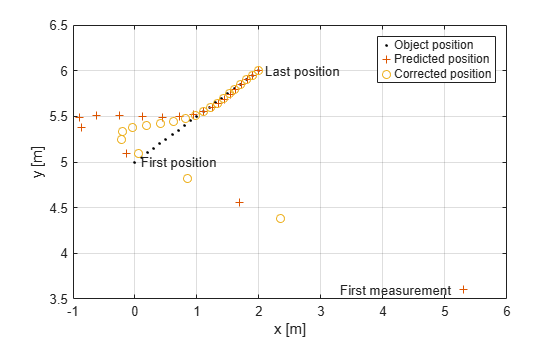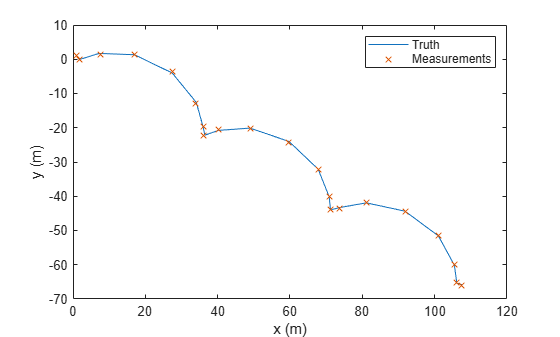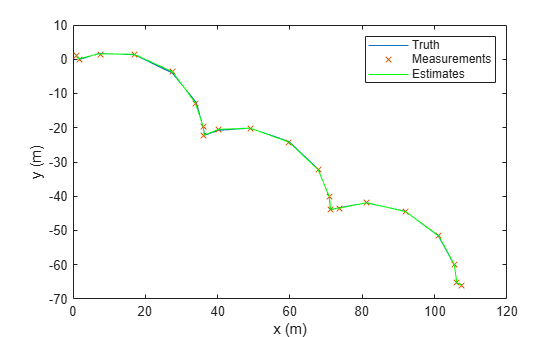# trackingKF

Linear Kalman filter for object tracking

## Description

A `trackingKF` object is a discrete-time linear Kalman filter used to track states, such as positions and velocities of target platforms.

A Kalman filter is a recursive algorithm for estimating the evolving state of a process when measurements are made on the process. The filter assumes the state-space model, including the state model and the measurement model, is linear. When the process noise and measurement noise are Gaussian and the motion model is linear, the Kalman filter is optimal. For a brief description of the linear Kalman filter algorithm, see Linear Kalman Filters.

You can use a `trackingKF` object in these ways:

• Set the `MotionModel` property to one of predefined state transition models. See the `MotionModel` property for details on these models.

• `"1D Constant Velocity"`

• `"1D Constant Acceleration"`

• `"2D Constant Velocity"`

• `"2D Constant Acceleration"`

• `"3D Constant Velocity"`

• `"3D Constant Acceleration"`

• Explicitly set the motion model. Set the `MotionModel` property to `"Custom"`, and then use the `StateTransitionModel` and `MeasurementModel` properties to specify the state transition matrix and measurement matrix, respectively. Optionally, you can specify control inputs by specifying the `ControlModel` property.

## Creation

### Syntax

``filter = trackingKF``
``filter = trackingKF("MotionModel",model)``
``filter = trackingKF(A,H)``
``filter = trackingKF(A,H,B)``
``filter = trackingKF(___,Name,Value)``

### Description

````filter = trackingKF` creates a discrete-time linear Kalman filter object for estimating the state of a 2-D, constant-velocity, moving object. The function sets the `MotionModel` property of the filter to `"2D Constant Velocity"`. ```
````filter = trackingKF("MotionModel",model)` sets the `MotionModel` property to a predefined motion model, `model`. In this case, the filter initializes the state as a double-precision zero vector based on the dimension of the motion model. The filter also configures the `MeasurementModel` property so that the measurement model returns position measurements.```
````filter = trackingKF(A,H)` specifies the `StateTransitionModel` and the `MeasurementModel` properties to `A` and `H`, respectively. The function sets the `MotionModel` property to `"Custom"`. ```

example

````filter = trackingKF(A,H,B)` sets the `ControlModel` property to the specified `B`. The function sets the `MotionModel` property to `"Custom"`. ```

example

````filter = trackingKF(___,Name,Value)` configures the properties of the Kalman filter by using one or more name-value arguments and any of the previous syntaxes. Any unspecified properties take default values. Enclose each property name in quotes.```

## Properties

expand all

Kalman filter state, specified as a real-valued M-element vector, where M is the size of the state vector. For information on the typical size of the state vector for each motion model, see the `MotionModel` property. If you specify the initial state as a scalar, the filter extends the state to an M-by-1 vector.

To use the filter with single-precision, floating-point variables, specify the `MootionModel` property as a predefined model and specify `State` as a single-precision vector variable. For example:

`filter = trackingKF("MotionModel","2D Constant Velocity","State",single([1; 2; 3; 4]))`

Example: `[200; 0.2; -40; -0.01]`

Data Types: `single` | `double`

State estimation error covariance, specified as a positive scalar or a positive-definite real-valued M-by-M matrix, where M is the size of the state vector. If you specify a scalar, the property value is the product of the specified scalar and an M-by-M identity matrix. The matrix represents the uncertainty in the state, and each diagonal element of the matrix represents the variance of the corresponding state component. The off-diagonal elements represent cross-covariance between different state components.

Example: `[20 0.1; 0.1 1]`

Data Types: `double`

Kalman filter motion model, specified as `"Custom"` or one of these predefined models:

• `"1D Constant Velocity"`

• `"1D Constant Acceleration"`

• `"2D Constant Velocity"`

• `"2D Constant Acceleration"`

• `"3D Constant Velocity"`

• `"3D Constant Acceleration"`

If you specify the property as one of the predefined motion models, the filter uses this state-space model:

`$\begin{array}{l}x\left(k+1\right)=A\left(k\right)x\left(k\right)+G\left(k\right)w\left(k\right)\\ z\left(k\right)=H\left(k\right)x\left(k\right)+v\left(k\right)\end{array}$`

where k is the discrete time step, x is the state, A is the state transition matrix, w is the process noise, G is the process noise gain matrix, H is the measurement matrix, v is the measurement noise, and z is the measurement. Note that the size of the gain matrix G is M-by-M/2, and the size of the process noise w is M/2, where M is the size of the state x.

Motion ModelState Vector xState Transition Matrix (A)Gain Matrix (G)
`"1D Constant Velocity"`

`[x;vx]`

`[1 dt; 0 1]`

`[dt^2/2; dt]`
`"2D Constant Velocity"`

`[x;vx;y;vy]`

Block diagonal matrix with the ```[1 dt; 0 1]``` block repeated for the x and y spatial dimensions

Kronecker product of ```kron(eye(2),[dt^2/2; dt])```

`"3D Constant Velocity"`

`[x;vx;y;vy;z;vz]`

Block diagonal matrix with the ```[1 dt; 0 1]``` block repeated for the x, y, and z spatial dimensions.

Kronecker product of ```kron(eye(3),[dt^2/2; dt])```

`"1D Constant Acceleration"`

`[x;vx;ax]`

```[1 dt dt^2/2; 0 1 dt; 0 0 1]```

`[dt^2/2; dt;1]`
`"2D Constant Acceleration"`

`[x;vx;ax;y;vy;ay]`

Block diagonal matrix with ```[1 dt dt^2/2; 0 1 dt; 0 0 1]``` blocks repeated for the x and y spatial dimensions

Kronecker product of ```kron(eye(2),[dt^2/2; dt; 1])```

`"3D Constant Acceleration"`

`[x;vx,ax;y;vy;ay;z;vz;az]`

Block diagonal matrix with the ```[1 dt 0.5*dt^2; 0 1 dt; 0 0 1]``` block repeated for the x, y, and z spatial dimensions

Kronecker product of ```kron(eye(3),[dt^2/2; dt; 1])```

In the table, `dt` is the time step specified in the `predict` object function. If you want process noise and measurement noise values different from the default values for the motion model, specify them in the `ProcessNoise` and `MeasurementNoise` properties, respectively.

If you specify `MotionModel` as `"Custom"`, you must specify a state transition model matrix A and a measurement model matrix H as input arguments to the Kalman filter. You can optionally specify a control model matrix, B, as well. When you specify a custom motion model, the filter uses this state-space model:

`$\begin{array}{l}x\left(k+1\right)=A\left(k\right)x\left(k\right)+B\left(k\right)u\left(k\right)+w\left(k\right)\\ z\left(k\right)=H\left(k\right)x\left(k\right)+v\left(k\right)\end{array}$`

where u is the control input. In this case, the size of the process noise w is M, where M is the size of the state x. You can specify the covariance of w using the `ProcessNoise` property, and specify the covariance of v using the `MeasurementNoise` property.

Data Types: `char` | `string`

State transition model between time steps, specified as a real-valued M-by-M matrix. M is the size of the state vector. In the absence of controls and noise, the state transition model predicts the state at a time step to the next time step.

Example: `[1 1; 0 1]`

#### Dependencies

To enable this property, set the `MotionModel` property to `"Custom"`.

Data Types: `single` | `double`

Control model, specified as an M-by-L matrix. M is the dimension of the state vector, and L is the number of controls or forces. The control model adds the effect of controls on the evolution of the state.

Note

To use a control model, you must specify this property when constructing the filter object. You cannot change the size of the control model matrix after creating the filter.

Example: `[.01 0.2]`

Data Types: `single` | `double`

Covariance of process noise, specified as a nonnegative scalar, a positive-semidefinite D-by-D matrix, or a positive-semidefinite M-by-M matrix. Process noise represents the uncertainty of state propagation, and the filter assumes the process noise to be zero-mean Gaussian white noise.

• When the `MotionModel` property is specified as one of the predefined motion models, specify the `ProcessNoise` property as a positive-semidefinite D-by-D matrix, where D is the number of dimensions of the target motion. For example, D = `2` for the `"2D Constant Velocity"` or the ```"2D Constant Acceleration"``` motion model.

In this case, if you specify the `ProcessNoise` property as a nonnegative scalar, then the scalar extends to the diagonal elements of a diagonal covariance matrix, of size D-by-D.

• When the `MotionModel` property is specified as `"Custom"`, specify the `ProcessNoise` property as a positive-semidefinite M-by-M matrix, where M is the size of the filter state. For example, M = `6` if you customize a 3-D motion model in which the state is (x, vx, y, vy, z, vz).

In this case, if you specify the `ProcessNoise` property as a nonnegative scalar, then the scalar extends to the diagonal elements of a diagonal covariance matrix, of size M-by-M.

Data Types: `single` | `double`

Measurement model from the state vector, specified as a real-valued N-by-M matrix, where N is the size of the measurement vector and M is the size of the state vector. The measurement model is a linear matrix that determines measurements from the filter state.

Note

You cannot change the size of the measurement model matrix after creating the filter.

Example: `[1 0.5 0.01; 1.0 1 0]`

Data Types: `single` | `double`

Covariance of the measurement noise, specified as a positive scalar or a positive-definite, real-valued N-by-N matrix, where N is the size of the measurement vector. If you specify this property as a scalar, the property value is the product of the specified scalar and the N-by-N identity matrix. Measurement noise represents the uncertainty of the measurement and the filter assumes measurement noise to be zero-mean Gaussian white noise.

Example: `0.2`

Data Types: `single` | `double`

Enable state smoothing, specified as `false` or `true`. Setting this property to `true` requires the Sensor Fusion and Tracking Toolbox™ license. When specified as `true`, you can:

• Use the `smooth` function, provided in Sensor Fusion and Tracking Toolbox, to smooth state estimates of the previous steps. Internally, the filter stores the results from previous steps to allow backward smoothing.

• Specify the maximum number of smoothing steps using the `MaxNumSmoothingSteps` property of the tracking filter.

Maximum number of backward smoothing steps, specified as a positive integer.

#### Dependencies

To enable this property, set the `EnableSmoothing` property to `true`.

Maximum number of out-of-sequence measurement (OOSM) steps, specified as a nonnegative integer.

• Setting this property to `0` disables the OOSM retrodiction capability of the filter object.

• Setting this property to a positive integer enables the OOSM retrodiction capability of the filter object. This option requires a Sensor Fusion and Tracking Toolbox license. Also, you cannot set the `MotionModel` property to `"Custom"`. When you set this property as N>1, the filter object saves the past state and state covariance history up to the last N+1 corrections. You can use the OOSM and the `retrodict` and `retroCorrect` (or `retroCorrectJPDA` for multiple OOSMs) object functions to reduce the uncertainty of the estimated state.

Increasing the value of this property increases the amount of memory that must be allocated for the state history, but enables you to process OOSMs that arrive after longer delays. Note that the effect of the uncertainty reduction using an OOSM decreases as the delay becomes longer.

## Object Functions

 `predict` Predict state and state estimation error covariance of linear Kalman filter `correct` Correct state and state estimation error covariance using tracking filter `correctjpda` Correct state and state estimation error covariance using tracking filter and JPDA `distance` Distances between current and predicted measurements of tracking filter `likelihood` Likelihood of measurement from tracking filter `clone` Create duplicate tracking filter `residual` Measurement residual and residual noise from tracking filter `smooth` Backward smooth state estimates of tracking filter `retrodict` Retrodict filter to previous time step `retroCorrect` Correct filter with OOSM using retrodiction `retroCorrectJPDA` Correct tracking filter with OOSMs using JPDA-based algorithm `initialize` Initialize state and covariance of tracking filter `tunableProperties` Get tunable properties of filter `setTunedProperties` Set properties to tuned values

## Examples

collapse all

Create a linear Kalman filter that uses a 2D constant velocity motion model. Assume that the measurement consists of the xy-location of the object.

Specify the initial state estimate to have zero velocity.

```x = 5.3; y = 3.6; initialState = [x;0;y;0]; KF = trackingKF('MotionModel','2D Constant Velocity','State',initialState);```

Create measured positions for the object on a constant-velocity trajectory.

```vx = 0.2; vy = 0.1; T = 0.5; pos = [0:vx*T:2; 5:vy*T:6]';```

Predict and correct the state of the object.

```for k = 1:size(pos,1) pstates(k,:) = predict(KF,T); cstates(k,:) = correct(KF,pos(k,:)); end```

Plot the tracks.

```plot(pos(:,1),pos(:,2),"k.",pstates(:,1),pstates(:,3),"+", ... cstates(:,1),cstates(:,3),"o") xlabel("x [m]") ylabel("y [m]") grid xt = [x-2, pos(1,1)+0.1, pos(end,1)+0.1]; yt = [y, pos(1,2), pos(end,2)]; text(xt,yt,["First measurement","First position","Last position"]) legend("Object position","Predicted position","Corrected position")```Specify a simulation time of 10 seconds with a time step of 1 second.

```rng(2021) % For repeatable results simulationTime = 20; dt = 1; tspan = 0:dt:simulationTime; steps = length(tspan);```

Specify the motion model as a 2-D constant velocity model with a state of [`x`; `vx`; `y`; `vy`]. The measurement is [`x`; `y`].

```A1D = [1 dt; 0 1]; A = kron(eye(2),A1D) % State transiton model```
```A = 4×4 1 1 0 0 0 1 0 0 0 0 1 1 0 0 0 1 ```
```H1D = [1 0]; H = kron(eye(2),H1D) % Measurement model ```
```H = 2×4 1 0 0 0 0 0 1 0 ```
```sigma = 0.2; R = sigma^2*eye(2); % Measurement noise covariance```

Specify a control model matrix.

```B1D = [0; 1]; B = kron(eye(2),B1D) % Control model matrix```
```B = 4×2 0 0 1 0 0 0 0 1 ```

Assume the control inputs are sinusoidal on the velocity components, `vx` and `vy`.

```gain = 5; Ux = gain*sin(tspan(2:end)); Uy = gain*cos(tspan(2:end)); U =[Ux; Uy]; % Control inputs```

Assuming the true initial state is [`1 1 1 -1`], simulate the system to obtain true states and measurements.

```initialState = [1 1 1 -1]'; % [m m/s m m/s] trueStates = NaN(4,steps); trueStates(:,1) = initialState; for i=2:steps trueStates(:,i) = A*trueStates(:,i-1) + B*U(:,i-1); end measurements = H*trueStates + chol(R)*randn(2,steps);```

Visualize the true trajectory and the measurements.

```figure plot(trueStates(1,:),trueStates(3,:),"DisplayName","Truth") hold on plot(measurements(1,:),measurements(2,:),"x","DisplayName","Measurements") xlabel("x (m)") ylabel("y (m)") legend```Create a `trackingKF` filter with a custom motion model. Enable the control input by specifying the control model. Specify the initial state in the filter based on the first measurement.

```initialFilterState = [measurements(1,1); 0; measurements(2,1); 0]; filter = trackingKF("MotionModel","Custom", ... "StateTransitionModel",A, ... "MeasurementModel",H, ... "ControlModel",B, ... "State",initialFilterState);```

Estimate states by using the `predict` and `correct` object functions.

```estimateStates = NaN(4,steps); estimateStates(:,1) = initialFilterState; for i = 2:steps predict(filter,U(:,i-1)); estimateStates(:,i) = correct(filter,measurements(:,i)); end```

Visualize the state estimates.

`plot(estimateStates(1,:),estimateStates(3,:),"g","DisplayName","Estimates");`Brown, R.G. and P.Y.C. Wang. Introduction to Random Signal Analysis and Applied Kalman Filtering. 3rd Edition. New York: John Wiley & Sons, 1997.

 Kalman, R. E. "A New Approach to Linear Filtering and Prediction Problems." Transaction of the ASME–Journal of Basic Engineering, Vol. 82, Series D, March 1960, pp. 35–45.

 Blackman, Samuel. Multiple-Target Tracking with Radar Applications. Artech House. 1986.